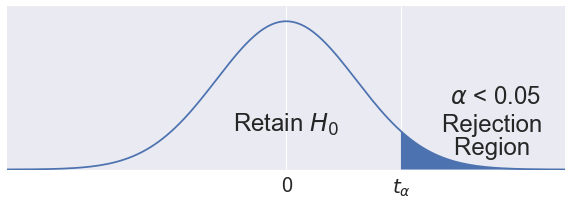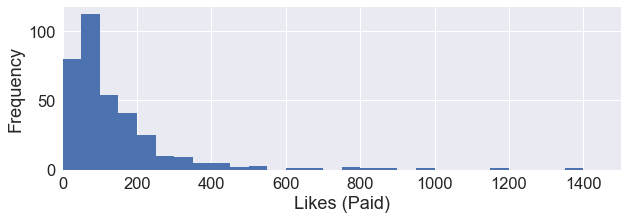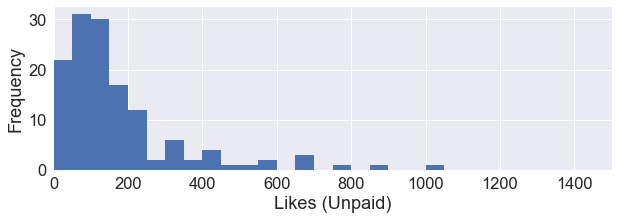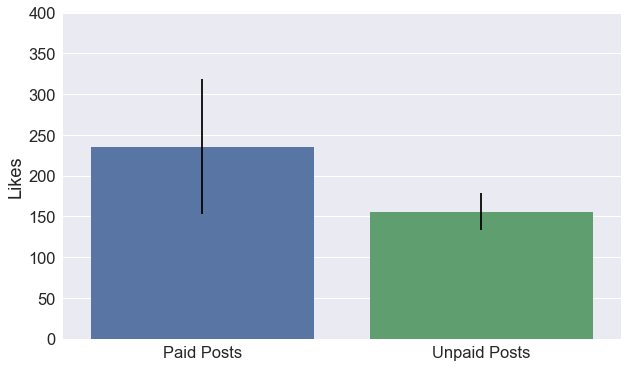Suppose that we are in the data science team for an orange juice company. In the meeting, the marketing team claimed that their new marketing strategy resulted in an increase of sales. The management team asked us to determine if this is actually true.

This is the data from January and February.

• Average Daily Sales in January = \$10,000, sample size = 31, variance = 10,000,000 • Average Daily Sales in February = \$12,000, sample size = 28, variance = 20,000,000

How do we know that the increase in daily orange juice sales was not due to random variation in data?

### The Null and Alternative Hypothesis¶

The amount of sales per day is not consistent throughout the month. The January data has a variance of 10,000,000 and a standard deviation of ~3162. On bad days, we would sell \$8,000 of orange juice. On good days, we would sell$14,000 of orange juice. We have to prove that the increase in average daily sales in February did not occur purely by chance.

The null hypothesis would be:

$H_0 : \mu_0 - \mu_1 = 0$

There are three possible alternative hypothesis:

1. $H_a : \mu_0 < \mu_1$
2. $H_a : \mu_0 > \mu_1$
3. $H_a : \mu_0 \ne \mu_1$

Where $\mu_0$ is the average daily sales in January, and $\mu_1$ is the average daily sales in February. Our null hypothesis is simply saying that there is no change in average daily sales.

If we are interested in concluding that the average daily sales has increased then we would go with the first alternative hypothesis. If we are interested in concluding that the average daily sales has decreased, then we would go with the second alternative hypothesis. If we are interested in concluding that the average daily sales changed, then we would go with the third alternative hypothesis.

In our case, the marketing department claimed that the sales has increased. So we would use the first alternative hypothesis.

### Type I and II Errors¶

We have to determine whether we accept or reject the null hypothesis. This could result in four different outcomes.

1. Retained the null hypothesis, and the null hypothesis was correct. (No error)
2. Retained the null hypothesis, but the alternative hypothesis was correct. (Type II error, false negative)
3. Rejected the null hypothesis, but the null hypothesis was correct. (Type I error, false positive)
4. Rejected the null hypothesis, and the alternative hypothesis was correct. (No error)

Hypothesis testing uses the same logic as a court trial. The null hypothesis(defendent) is innocent until proven guilty. We use data as evidence to determine if the claims made against the null hypothesis is true.

### Significance Level¶

In order to come to a decision, we need to know if the February data is statistically significant. We would have to calculate the probability of finding the observed, or more extreme data assuming that the null hypothesis, $H_0$ is true. This probability is known as the p-value.

If this probability is high, we would retain the null hypothesis. If this probability is low, we would reject the null hypothesis. This probability threshold known as the significance level, or $\alpha$. Many statisticians typically use $\alpha$ = 0.05.

To visualize this using the probabiliy distribution, recall that we've chosen to prove that $\mu_0 < \mu_1$. This is called a right-tailed test.

In :
import matplotlib.pyplot as plt
import numpy as np
import math
import seaborn as sns
from scipy.integrate import simps
%matplotlib inline

#The Gaussian Function
def g(x):
return 1/(math.sqrt(1**math.pi))*np.exp(-1*np.power((x - 0)/1, 2)/2)

fig = plt.figure(figsize=(10,3))
x = np.linspace(-300, 300, 10000)
sns.set(font_scale=2)

#Draws the gaussian curve
ax.plot(x, g(x))
ax.set_ylim(bottom = 0, top = 1.1)
ax.set_xlim(left = -4, right = 4)
ax.set_yticks([])
plt.xticks([0, 1.645],
[0, r'$t_\alpha$']
)

#Fills the area under the curve
section = np.arange(1.645, 300, 1/2000)
ax.fill_between(section, g(section))

#Calculates the area under the curve using Simpson's Rule
x_range = np.linspace(1.645, 300, 2000)
y_range = g(x_range)
area_total = simps(g(x), x)
area_part = simps(y_range , x_range)
percent_data = np.round((area_part/area_total), 2)
ax.annotate(r'$\alpha$ < {}'.format(percent_data), xy=(3, 0.45), ha='center')
ax.annotate('Rejection '.format(1-percent_data), xy=(3, 0.26), ha='center')
ax.annotate('Region '.format(1-percent_data), xy=(3, 0.1), ha='center')
ax.annotate('Retain $H_0$', xy=(0, 0.26), ha='center')
plt.show()We don't know where the data from February is on this distribution. We'll still to calculate the p-value to determine if we are in the rejection region. The p-value can only answer this question: how likely is February data, assuming that the null hypothesis is true? If we do end up with a p-value less than 0.05, then we will reject the null hypothesis.

#### Other Cases:¶

If our alternative hypothesis was $\mu_0 > \mu_1$, then we would have to use a left-tailed test, which is simply the flipped veresion of the right-tailed test.

If our alternative hypothesis was $\mu_0 \ne \mu_1$, then we would have to use a two-tailed test, which is both the left and right tailed test combined with $\alpha$ = 0.025 on each side.

### The Welch's t-test¶

One way to tackle this problem is to calculate the probability of finding February data in the rejection region using the Welch's t-test. This version of the t-test can be used for equal or unequal sample sizes. In addition, this t-test can be used for two samples with different variances. This is often praised as the most robust form of the t-test. However, the Welch's t-test assumes that the two samples of data are independent and identically distributed.

The t-score can be calculated using the following formula:

$$t_{score} = \frac {\bar {X}_1 - \bar {X}_2} {s_{Welch}}$$

$$s_{Welch} = \sqrt{\frac{s^2_1} {n_1}+\frac{s^2_2} {n_2}}$$

The degrees of freedom can be calculated using the following formula:

$$DoF = \frac{\bigg({\frac{s^2_1} {n_1}+\frac{s^2_2} {n_2}}\bigg)^2} {\frac{({s^2_1}/{n_1})^2}{n_1-1} + \frac{({s^2_2}/{n_2})^2}{n_2-1}}$$

Where $\bar {X}$ is the sample average, $s$ is the variance, and $n$ is the sample size. With the degrees of freedom and the t-score, we can use a t-table or a t-distribution calculator to determine the p-value. If the p-value is less than the significance level, then we can conclude that our data is statistically significant and the null hypothesis will be rejected.

We could plug in every number into python, and then looking up a t-table. But it is easier to just use the scipy.stats module. Click here for the link to the documentation.

In :
from scipy import stats

t_score = stats.ttest_ind_from_stats(mean1=12000, std1=np.sqrt(10000000), nobs1=31, \
mean2=10000, std2=np.sqrt(20000000), nobs2=28, \
equal_var=False)
t_score

Out:
Ttest_indResult(statistic=1.9641226483541647, pvalue=0.055312326250267031)

From the Welch's t-test we ended up with a p-value of 0.055. Scipy calculates this value based on the two tailed case. If we just want the p-value of the right-tail, we can divide this value by 2. This means that the probability that there is a ~2.57% chance of finding the observed values from February given the data from January. We should reject the null hypothesis.

### The Welch's t-test with Facebook Data¶

Let's try using real data this time. I've taken data from UCI's machine learning repository. Click here for the link to the documentation and citation. In summary, the data is related to 'posts' published during the year of 2014 on the Facebook's page of a renowned cosmetics brand.

Suppose our cosmetics company wants more skin in the digital marketing game. We are interested in using Facebook as a platform to advertise our company. Let's start with some simple data exploration.

In :
import pandas as pd

Out:
0 139441 Photo 2 12 4 3 0.0 2752 5091 178 109 159 3078 1640 119 4 79.0 17.0 100
1 139441 Status 2 12 3 10 0.0 10460 19057 1457 1361 1674 11710 6112 1108 5 130.0 29.0 164
2 139441 Photo 3 12 3 3 0.0 2413 4373 177 113 154 2812 1503 132 0 66.0 14.0 80
3 139441 Photo 2 12 2 10 1.0 50128 87991 2211 790 1119 61027 32048 1386 58 1572.0 147.0 1777
4 139441 Photo 2 12 2 3 0.0 7244 13594 671 410 580 6228 3200 396 19 325.0 49.0 393

#### Exploring 'Paid' and 'Unpaid' Facebook Posts¶

We are interested in knowing the amount of likes a 'Paid' post gets vs. an "Unpaid" post. Let's start with some histograms and descriptive statistics.

In :
unpaid_likes = data[data['Paid']==0]['like']
unpaid_likes = unpaid_likes.dropna()
sns.set(font_scale=1.65)
fig = plt.figure(figsize=(10,3))
ax=unpaid_likes.hist(range=(0, 1500),bins=30)
ax.set_xlim(0,1500)

plt.xlabel('Likes (Paid)')
plt.ylabel('Frequency')
plt.show()

print('sample_size: {}'.format(unpaid_likes.shape))
print('sample_mean: {}'.format(unpaid_likes.mean()))
print('sample_variance: {}'.format(unpaid_likes.var()))sample_size: 359
sample_mean: 155.84679665738162
sample_variance: 48403.23623970993

In :
paid_likes = data[data['Paid']==1]['like']
fig = plt.figure(figsize=(10,3))
ax=paid_likes.hist(range=(0, 1500),bins=30)
ax.set_xlim(0,1500)

plt.xlabel('Likes (Unpaid)')
plt.ylabel('Frequency')
plt.show()

print('sample_size: {}'.format(paid_likes.shape))
print('sample_mean: {}'.format(paid_likes.mean()))
print('sample_variance: {}'.format(paid_likes.var()))sample_size: 139
sample_mean: 235.6474820143885
sample_variance: 247175.07048274425


#### The Confidence Interval¶

We can also explore by the data by calculating the confidence interval. This is a range of values that is likely to contain the value of an unknown population mean based on our sample mean. In this case, I've split the data into two categories, 'Paid' and 'Unpaid'. As a result, the population mean will be calculated with respect to each categories.

Here are the assumptions:

• The data must be sampled randomly.
• The sample values must be independent of each other.
• The sample size must be sufficiently large to use the Central Limit Theorem. Typically we want to use N > 30.

We can calculate this interval by multiplying the standard error by the 1.96 which is the score for a 95% confidence. This means that we are 95% confident that the population mean is somewhere within this interval.

In other words, if we take many samples and the 95% confidence interval was computed for each sample, 95% of the intervals would contain the population mean.

In :
paid_err = 1.96*(paid_likes.std())/(np.sqrt(paid_likes.shape))
unpaid_err = 1.96*(unpaid_likes.std())/(np.sqrt(unpaid_likes.shape))

x = ['Paid Posts', 'Unpaid Posts']
y = [paid_likes.mean(), unpaid_likes.mean()]
fig = plt.figure(figsize=(10, 6))
ax = sns.barplot(x=x, y=y, yerr=[paid_err, unpaid_err])
ax.set_ylim(0, 400)
plt.ylabel('Likes')
plt.show()From the chart above, we can see that the error bars for 'Paid' posts and "Unpaid' posts have an overlapping region. We can also see that the sample mean of 'likes' in paid posts was higher than the sample mean of 'likes' in unpaid posts. We need to determine if the data we have is statistically significant and make sure that our results did not occur purely by chance.

The null hypothesis would suggest that paying for advertisements does not increase the amount of likes.

$$H_0 : \mu_0 = \text{139 likes}$$

The alternative hypothesis would suggest that paying for advertisements does increase the amount of likes.

$$H_a : \mu_1 > \text{139 likes}$$

We can come to a decision using the right-tailed Welch's t-test again. This time, we'll calculate the p-value in using the formulas in the previous section instead of the scipy module.

In :
s_welch = np.sqrt(paid_likes.var()/paid_likes.shape + unpaid_likes.var()/unpaid_likes.shape)
t=(paid_likes.mean()-unpaid_likes.mean())/s_welch
print('t-value: {}'.format(t))

t-value: 1.824490721115131

In :
df_num = (paid_likes.var()/paid_likes.shape + unpaid_likes.var()/unpaid_likes.shape)**2
df_dem = (
(paid_likes.var()/paid_likes.shape)**2/(paid_likes.shape-1)) + \
(unpaid_likes.var()/unpaid_likes.shape)**2/(unpaid_likes.shape-1)
df = df_num/df_dem
print('degrees of freedom: {}'.format(df))

degrees of freedom: 159.3668015083367


Using the t-table here. We only need a t-score of 1.658 and degrees of freedom of at least 120 to get a p-value of 0.05.

Next, we'll use scipy again to determine the exact p-value.

In :
t_score = stats.ttest_ind_from_stats(paid_likes.mean(), paid_likes.std(), paid_likes.shape, \
unpaid_likes.mean(), unpaid_likes.std(), unpaid_likes.shape, \
equal_var=False)
t_score

Out:
Ttest_indResult(statistic=1.8244907211151311, pvalue=0.069950722795108722)

From the Welch's t-test we ended up with a two-tailed p-value of ~0.07, or ~0.035 for a one-tail test. We will reject the null hypothesis, and accept that Facebook advertisements does have a positive effect on the number "likes" on a post.

Notes:

1. The p-value does NOT give us the probability that the null hypothesis is false. We also don't know the probability of the alternative hypothesis being true.
2. The p-value does not indicate the magnitude of the observed effect, we can only conclude that the effects were positive.
3. The 0.05 p-value is just a convention to determine statistical significance.
4. We can not make any predictions about the repeatability of the t-test, we could get completely different p-values based on the sample size.

The files used for this article can be found in my GitHub repository.